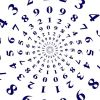#### You may also like### Curvy Equation

This problem asks you to use your curve sketching knowledge to find all the solutions to an equation.### Digital Equation

Can you find a three digit number which is equal to the sum of the hundreds digit, the square of the tens digit and the cube of the units digit?### Euler's Totient Function

How many numbers are there less than $n$ which have no common factors with $n$?

# Proper Factors

##### Age 16 to 18Challenge Level

proper factor of an integer $N$ is a positive integer, not $1$ or $N$, that divides $N$.

1. Show that $3^2\times 5^3$ has exactly $10$ proper factors. Determine how many other integers of the form $3^m\times5^n$ (where $m$ and $n$ are integers) have exactly $10$ proper factors.
It might be easier to start by considering the total number of factors, rather than just the proper factors. You can also consider some simpler cases first to get a "feel" for the problem. How many factors are there of $3$, $3 \times 5$ and $3^2 \times 5$?

2. Let $N$ be the smallest positive integer that has exactly $426$ proper factors. Determine $N$, giving your answer in terms of its prime factors.
One way to get some insights into how to tackle this part is to consider a simpler case first. Can you find the smallest positive integer which has exactly $10$ proper factors?  Note that we don't have to restrict ourselves to numbers of the form $3^m \times 5^n$, we could have powers of $2$, $7$ etc.

STEP Mathematics I, 2009, Q1. Question reproduced by kind permission of Cambridge Assessment Group Archives. The question remains Copyright University of Cambridge Local Examinations Syndicate ("UCLES"), All rights reserved.